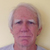If I had to build a stem wall for a manufactured home that was 20′ x 60′ what and how much would I need| Posted in General Questions on

a concrete stem wall for a manufactured home that is 20′ x 60′ – what supplies and how much of each would be neededJoin the leading community of building science experts

Replies

1.Expert Member
| | #1

Golfson,

You either need to learn how to do the materials takeoff, or hire a builder to do one for you.

2.| | #2

so in other words you do not know the answer either - I thought maybe a formula would help me for the material takeoff since I kinow the sq ft

3.| | #3

so in other words you do not know the answer either - I thought maybe a formula would help me for the material takeoff since I kinow the sq ft

4.| | #4

Golfson: In order to answer the question, we'd need to know the other dimensions. How wide and how deep. Depth is climate specific. Multiply linear feet(in this case, 160) by depth in feet, by width in feet (typically 8" or . 667'). The result is in cubic feet. Divide by 27 and you get cubic yards, the usual concrete measure.
Malcolm tried to gently suggest that if you can't figure this out, maybe you need a contractor.

5.• |
• |
• |
• |